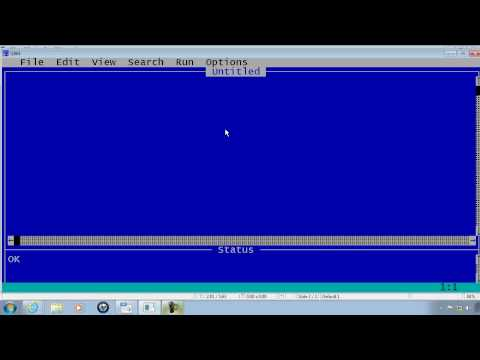## String Functions

Subject: Computer Science

#### Overview

String functions are used to manipulate strings. A string can defined as set of alphanumeric characters. This note provides an information about String Functions .
##### String Functions

String functions are used to manipulate strings. A string can defined as set of alphanumeric characters.

Some of the strings manipulating functions are as follows:

• LEFT$Function: It is a string function that returns specific number of characters from left side to supplied string and returns the value. • RIGHT$ Function: It is a string function that returns specific number of characters from right side to supplied string and returns the value.
• MID$Function: IT returns the specified number of characters from the specified location of a string. • UCASE$ Function: It returns a string expression with all letters in uppercase.
• LCASE$Function: It returns a string expression with all letters in lowercase. • CHR$ Function: IT retrieves the single characters and returns a character of the corresponding ASCII code in decimal.
• STR$Function: It converts a numeric expression into its string data returns the string value. • LTRIM$ Function: It removes trailing blank spaces from the left side of the string expression.
• SPACE$Function: It returns a number of spaces. • INSTR Function: It returns the position of first occurrence of a string in another string. • STRING$ Function: It is used with a PRINT statement that displays a particular character a specified number of times.
• INKEY$Function: It reads a character from the keyboard and returns the character. • INPUT$ Function: It returns a string of characters read from the specified file.
• DATE$Function: It returns the current system date of computer in string format in the form of MM/DD/YYYY. • TIME$ Function: It returns the current system time of computer in string format in the form of HH:MM:SS.##### Things to remember
• String functions are used to manipulate strings.
• LEFT$Function returns specific number of characters from left side to supplied string and returns the value. • RIGHT$ Function returns specific number of characters from right side to supplied string and returns the value.
• MID$Function returns the specified number of characters from the specified location of a string. • It includes every relationship which established among the people. • There can be more than one community in a society. Community smaller than society. • It is a network of social relationships which cannot see or touched. • common interests and common objectives are not necessary for society. ##### Videos for String Functions##### QBasic Tutorial 22 - String Manipulation - QB64 ##### Questions and Answers DECLARE SUB KINS(A$)
CLS
A$= "NEPAL" CALL KINS(A$)
END
SUB KINS(A$) FOR I = LEN(A$) to 1 Step-1
PRINT LEFT$(A$,1)
NEXT I
END SUB
DECLARE SUB KINS(A$) CLS A$= "Nepal"
CALL KINS
END
SUB KINS (A$) FOR I = 1 to LEN (A$)
PRINT RIGHT$(A$,1)
NEXT I
END SUB

DECLARE SUB KINS(A$) CLS CALL KINS(A$)
END
SUB KINS(A$) FOR I = 1 to LEN(A$)
PRINT MID$(A$,I,1)
NEXT I
END SUB
Using FUNCTION Procedure:
DECLARE FUNCTION Pal(A$) CLS INPUT"Enter any string";A CALL Pal(A$)
END
FUNCTION Pal(A$) FOR I = LEN(A$) to 1 Step-1
B$= B$+MID$(A$,I,1)
NEXT I
IF A$= B$ THEN
A$= "It is palindrome" ELSE A$="It is not palindrome"
END IF
END FUNCTION
DECLARE SUB Alter(A$) CLS INPUT"Enter any string";A$
CALL Alter(A$) END SUB Alter(A$)
FOR I = 1 to LEN(A$) C$= MID$(A$,I,1)
IF I MOD 2 = 1 THEN
B$=B$+ Ucase$(C$)
ELSE
B$=B$+ Lcase$(C$)
ENDOF
NEXT I
PRINT"Alternate character is";B$END SUB DECLARE FUNCTION Sentence(A$)
CLS
PRINT Sentence(A$) END FUNCTION Sentence(A$)
FOR I = 1 to LEN(A$) B$= Ucase$(MID$(A$,I,1) IF B$= "A" THEN C= C+1
NEXT I
Sentence = C
END FUNCTION